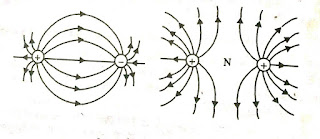Electric Field Intensity: Definition,Properties and Example- Electrical Diary - Electrical Diary -->

# What is Electric Field?

The region around a charge or group of charges in which a force is experienced by another test charge is called Electric Field. it is a region around a charge in which an external charge of any nature always experienced a force. The nature of force may be Either attractive or repulsive.it will depend on the nature of the charge which has created the electric field and also the nature of the external charge.

## What is Electric Field Intensity?

The concept of Electric Field Intensity is used to measure the strength of the electric field at a point in the region of the electric field. it is defined as the force experienced by a positive test charge having one column charge. Sometimes it is called electric field strength.it is a vector quantity. its SI unit is newton per Columb.

### Physical Significance of Electric Field

The concept of the Electric field is used to describe the electrical environment around a charge or group of charges or system of a charge. The electric field describes how much force is experienced by a positive unit charge if this a positive test charge is put in the region of the electric field without disturbing the system of the charge. In physics, the concept of field is used to represent a physical quantity that can be defined at every point of space and it may vary from point to point in space. The electric field is a vector quantity because it is defined with force and force is a vector quantity.

### Formula and Unit of Electric Field Intensity

Since Electric Field Intensity is defined as the Ratio of Force experienced per unit of charge then it may be given as
As from the formula, it is clear that Electric Field is the ratio of two physical quantity force and Charge, if Force is measured in Newton (N) and Charges in Coulomb (C) then the Unit for Electric Field Can be easily Derive like this

In short Unit of Electric Field Intensity can be written as N/C.

### Electric Field Lines

if a charge is kept in the electric field and it is free to move then it will start moving in the direction of force experienced by the Electric Field. In the Electric field, the path followed by a free charge is called the electric line of force.

Hence electric field lines are imaginary smooth curves on which a free and isolated charge move. At any instant tangent to the curve give the direction of the electric field. In the electric field region, the direction of the electric field is given by electric field lines.

The concept of Electric field lines is first of all given by Michael Faraday to describe the behavior of the Electric field in a given region. According to Michael FaradayElectric Field lines, originate from a positive charge and terminate on a negative charge. Electric field lines can be represented by arrows according to the figure given belowFig (1)Fig (2)
In the above-given figure(1) electric field lines are originating from a positive charge and terminate on a negative charge for a two charge system. But in figure (2) Electric field lines are originating from Positive charge and Electric Field lines are terminating from infinity to negative charge.

### The direction of Electric Field lines

While dealing with the Electric field it becomes important to determine the direction of the Electric Field because it is a vector quantity. The direction of the Electric field may be in any direction along three axes of the Coordinate system. But in the general, the direction of Electric Field lines is always away from the Positive charge.

### Properties of Electric Field Lines

• Electric Field lines originate from a positive charge and terminate on a negative charge.
• At any point in the electric field tangent to field lines gives the direction of the electric field at any instant of time.
• Two field lines of a single source of charge never intersect each other because at the point of intersection it is possible to draw two tangents, which will give two directions of electric field lines which is not possible.
• Electric field lines in a uniform magnetic field are parallel to each other at an equal distance from each other.
• Electric field lines originate perpendicular to the surface of a charged conductor.